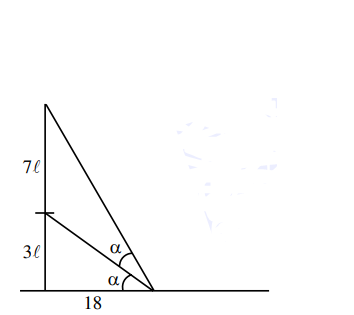# A vertical pole fixed to the horizontal ground is divided`
Question:

A vertical pole fixed to the horizontal ground is divided in the ratio $3: 7$ by a mark on it with lower part shorter than the upper part. If the two parts subtend equal angles at a point on the ground $18 \mathrm{~m}$ away from the base of the pole, then the height of the pole (in meters) is:

1. $12 \sqrt{15}$

2. $12 \sqrt{10}$

3. $8 \sqrt{10}$

4. $6 \sqrt{10}$

Correct Option: , 2

Solution:Let height of pole $=10 \ell$

$\tan \alpha=\frac{3 \ell}{18}=\frac{\ell}{6}$

$\tan 2 \alpha=\frac{10 \ell}{18}$

$\frac{2 \tan \alpha}{1-\tan ^{2} \alpha}=\frac{10 \ell}{18}$

use $\tan \alpha=\frac{\ell}{6} \Rightarrow \ell=\sqrt{\frac{72}{5}}$

height of pole $=10 \ell=12 \sqrt{10}$